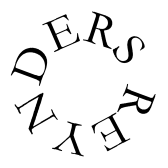# SOGONEKU abdoul doumbia

O = tone

oo = flam

S = slap

B = bass

`*all single tones are muffed in the rhythm.....`

1 - - 2 - - 3 - - 4 - - 5 - - 6

flam muff muff flam

`oo     O S        S        oo     B B      S B     Djembe 1`

flam muff muff flam muff muff

`oo    O S         S        oo     O S        S     Djembe 2`

1 - - 2 - - 3 - - 4 - - 5 - - 6

O O O O O O Dun Dun 1

`                       O O O O                     Sangban`

SÖKÖ

Sökö is a rhythm of the Komanko-people in the Faranah-region. Nowadays it's played often in the whole North of Guinea. The rhythm accompanies the dance of the bilakoro (they who are not circumcised yet). In some regions it's played during the months before cicumcision, elsewhere it's played the day before initiation after the heads of the bilakoro have been shaved en presents have been given.

Sökö / WAP-pages / Paul Nas / Last changed at 23-01-1999

Sangban

`C . . C . . O O . O O . C . . C . . C . . O O .`
`x x . x x . x x . x x . x x . x x . x x . x x .`

Kenkeni; Faranah-style

`. . . O O . . . . O O . . . . O O . . . . O O .`
`x x . x x . x x . x x . x x . x x . x x . x x .`

Dun Dun; Faranah-style

`O . . . . O O . O O . O O . . . . . . . O O . O`
`x . x x . x x . x x . x x . x x . x x . x x . x`

Kenkeni; Hamana-style

`O . . O . . O . . O . . O . . O . . O . . O . .`
`x x . x x . x x . x x . x x . x x . x x . x x .`

Dundun; Hamana-style

`O . . . O O . O . O . O O . . . . . . . O O . O`
`x . x . x x . x . x . x x . x x . x x . x x . x`

Sangban; alternative

`C . C . C . O O . O O . C . C . C . C . . O O .`
`x . x . x . x x . x x . x . x . x . x x . x x .`

Djembé 1

`S . S S T T S . S S T T S . S S T T S . S S T T`
`r . r l r l r . r l r l r . r l r l r . r l r l`

Djembé 2

`S . T S t t S . T S t t S . T S t t S . T S t t`
`r . r l . . r . r l . . r . r l . . r . r l . .`

Djembé 3

`S T T S . S S T T S . S S T T S . S S T T S . S`
`r l r l . l r l r l . l r l r l . l r l r l . l`

Solo accompagnement 1

`B T T . S . b S . b S . B T T . S . b S . b S .`
`r l r . r . . l . . r . r l r . r . . l . . r .`